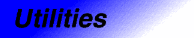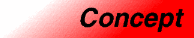# LessThanComparableCategory: utilities Component type: concept

## Description

A type is LessThanComparable if it is ordered: it must be possible to compare two objects of that type using `operator<`, and `operator<` must be a partial ordering.

## Notation

 `X` A type that is a model of LessThanComparable `x`, `y`, `z` Object of type `X`

## Definitions

Consider the relation `!(x < y) && !(y < x)`. If this relation is transitive (that is, if `!(x < y) && !(y < x) && !(y < z) && !(z < y)` implies `!(x < z) && !(z < x)`), then it satisfies the mathematical definition of an equivalence relation. In this case, `operator<` is a strict weak ordering.

If `operator<` is a strict weak ordering, and if each equivalence class has only a single element, then `operator<` is a total ordering.

## Valid expressions

Name Expression Type requirements Return type
Less `x < y`   Convertible to `bool`
Greater `x > y`   Convertible to `bool`
Less or equal `x <= y`   Convertible to `bool`
Greater or equal `x >= y`   Convertible to `bool`

## Expression semantics

Name Expression Precondition Semantics Postcondition
Less `x < y` `x` and `y` are in the domain of `<`
Greater `x > y` `x` and `y` are in the domain of `<` Equivalent to `y < x` 
Less or equal `x <= y` `x` and `y` are in the domain of `<` Equivalent to `!(y < x)` 
Greater or equal `x >= y` `x` and `y` are in the domain of `<` Equivalent to `!(x < y)` 

## Invariants

 Irreflexivity `x < x` must be false. Antisymmetry `x < y` implies !(y < x)  Transitivity `x < y` and `y < z` implies `x < z` 

• int

## Notes

Copyright © 2006-2007 Adobe Systems Incorporated.

Use of this website signifies your agreement to the Terms of Use and Online Privacy Policy.

Search powered by Google